Science Journal of Applied Mathematics and Statistics
Volume 3, Issue 4, August 2015, Pages: 171-176

Multivariate Outlier Detection Using Independent Component Analysis

Md. Shamim Reza, Sabba Ruhi

Department of Mathematics, Pabna University of Science & Technology, Pabna, Bangladesh(Md. S. Reza)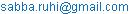(S. Ruhi)

Md. Shamim Reza, Sabba Ruhi. Multivariate Outlier Detection Using Independent Component Analysis. Science Journal of Applied Mathematics and Statistics. Vol. 3, No. 4, 2015, pp. 171-176. doi: 10.11648/j.sjams.20150304.11

Abstract: The recent developments by considering a rather unexpected application of the theory of Independent component analysis (ICA) found in outlier detection, data clustering and multivariate data visualization etc. Accurate identification of outliers plays an important role in statistical analysis. If classical statistical models are blindly applied to data containing outliers, the results can be misleading at best. In addition, outliers themselves are often the special points of interest in many practical situations and their identification is the main purpose of the investigation. This paper takes an attempt a new and novel method formultivariate outlier detection using ICA and compares with different outlier detection techniques in the literature.

Keywords: Kurtosis, Outlier, Independent Component Analysis, Normality

1. Introduction

Independent component analysis (ICA) is a Statistical and computational technique in which the goal is to find a linear projection of the data that the source signals or components are statistically independent or as independent as possible. It is probably fair to say that in the last 10 years, ICA has become a standard tool in machine learning and signal processing . Among its numerous applications, ICA is the most natural tool for BSS  in instantaneous linear mixtures when the source signals are assumed to be independent. The plausibility of the statistical independence assumption in a wide variety of fields, including telecommunications, finance and biomedical engineering, helps explain the arousing interest in this research area witnessed over the last two decades. The above numerious applications of ICA suffer from the curse of outlier and dimensionality, hence, outlier identification is very tedious such large dimension. Even though there are existing methodologies attending to high dimensionality and outlier detection problems, most of them are computationally intensive and time consuming. The detection of outliers is an important problem in model building, inference and multivariate data analysis. Indeed, the presence of outliers, even in small quantity, can lead to biased estimation of the parameters, to a misspecification of the model and to inappropriate predictions.

Many methods for outlier detection try to detect outliers .Outlier detection is carried out through the use of Principal Components Analysis (PCA) . PCA is a dimension reduction procedure where some of the variables are highly correlated with each other. If this is to be used in a contaminated data, the nature of the estimated principal components may behave differently, implemented the principal components as a multivariate outlier detection method . The basis for multivariate outlier detection is the Mahalanobis distance. The standard method for multivariate outlier detection is robust estimation of the parameters in the Mahalanobis distance and the comparison with a critical value of the Chi-Square distribution . In this article, we will begin a general description of outlier detection, briefly describing the most popular methods of multivariate outlier detection such as PCA, ICA and proposed ICA on PCA. In this article, we take an attempt to visualize multivariate data using independent component analysis as well as to detect outlier and comparing their performance among other outlier detection method that found best in the literature. We briefly discuss univariate and multivariate outlier in section II. Section III and section IV we describe the methods of multivariate outlier detection PCA and ICA. In section V and VI we discuss classical and quantile measures of kurtosis estimators and proposed flowchart to detect outlier. We then apply quantile kurtosis estimator in sorting independents components to detect outlier. The final section gives conclusion.

2. Outlier

In statistics, an outlier refers to a case that deviates to a notable extent from the typical range or pattern of observations exhibited for other cases. Outliers themselves are often the special points of interest in many practical Situations and their identification is the main purpose of the investigation. An exact definition of an outlier often depends on hidden assumptions regarding the data structure and the applied detection method. Yet, some definitions are regarded general enough to cope with various types of data and methods. Hawkins  defines an outlier as an observation that deviates so much from other observations as to arouse suspicion that it was generated by a different mechanism. Barnet and Lewis  indicate that an outlying observation, or outlier, is one that appears to deviate markedly from other members of the sample in which it occurs, similarly, Johnson  defines an outlier as an observation in a data set which appears to be inconsistent with the remainder of that set of data.

A univariate outlier is a data point that consists of an extreme value on one variable. A multivariate outlier is a combination of unusual scores on at least two variables. Both types of outliers can influence the outcome of statistical analyses. Outliers exist for four reasons. Incorrect data entry can cause data to contain extreme cases. A second reason for outliers can be failure to indicate codes for missing values in a dataset. Another possibility is that the case did not come from the intended sample. And finally, the distribution of the sample for specific variables may have a more extreme distribution than normal. In many parametric statistics, univariate and multivariate outliers must be removed from the dataset. When looking for univariate outliers for continuous variables, standardized values (z scores) can be used. For continuous variables, univariate outliers can be considered standardized cases that are outside the absolute value of 3.29. However, caution must be taken with extremely large sample sizes, as outliers are expected in these datasets. Once univariate outliers have been removed from a dataset, multivariate outliers can be assessed for and removed. Multivariate outliers can be identified with the use of Mahalanobis distance, which is the distance of a data point from the calculated centroid of the other cases where the centroid is calculated as the intersection of the mean of the variables being assessed. Each point is recognized as an X, Y combination and multivariate outliers lie a given distance from the other cases. Some common multivariate techniques such as PCA, and recently developed ICA describe the next section.

3. Principal Component Analysis

Principal component analysis or PCA is one of the key tools in multivariate statistical analysis and is often used to reduce the dimension of data for easy exploration. As a multivariate analysis technique for dimension reduction, it aims to compress the data without losing much information the original data contains. The process of how PCA is done here is based on Johnson, R. . It is concerned with explaining the variance-covariance structure of a set of variables through a few new variables. All principal components are particular linear combinations of the p random variables with three important properties which are:

i.        The principal components are uncorrelated.

ii.        The first principal component has the highest variance; the second principal component has the second highest variance, and so on.

iii.        The total variation in all the principal components combined is equal to the total variation in the original variables.

Mathematically,

Let X and Y are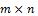matrices related by a linear transformation P. X is the original recorded data set and Y is a re-representation of that data set.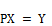(1)

Equation 1 represents a change of basis and thus can have many interpretations.

1. P is a matrix that transforms X into Y.

2. Geometrically, P is a rotation and a stretch which again transforms X into Y.

3. The rows of P,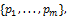are a set of new basis vectors for expressing the columns of X. Where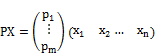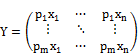We can note the form of each column of Y. The new variable Y is linear combination of original variables X.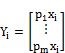The first PC is the linear combination of the variables that explains the greatest amount of the total variation in x. The second PC is the linear combination of the variables that explains the next largest amount of variation and is uncorrelated with the first PC, and so on. If the first few (say, three) components contain most of the total variation (say, 85%), then the original variables can be replaced by these components without too much loss of variance information. The principal components are computed from an eigen analysis of the covariance matrix or the correlation matrix, but results from the covariance matrix and the correlation matrix are usually not the same. If the variables are measured on scales with widely different ranges or if the units of measurement are not commensurate, it is better to perform PCA on the correlation matrix. The observations that are outliers with respect to the first few principal components or the major principal components usually correspond to outliers on one or more of the original variables. On the other hand, the last few principal components or the minor principal components represent linear functions of the original variables with the minimal variance. The minor principal components are sensitive to the observations that are inconsistent with the correlation structure of the data, but are not outliers with respect to the original variables.

4. Independent Component Analysis

Independent component analysis (ICA) is a statistical method used to discover hidden factors (sources or features) from a set of measurements or observed data such that the sources are maximally independent. The ICA algorithms are able to separate the sources according to the distribution of the data. Independent component analysis (ICA) , and projection pursuit (PP) , are closely related techniques, which try to look for interesting directions (projections) in the data. To achieve separation of mixed data into independent components ICA exploits the independence between the sources in order to achieve their separation from mixed data. In order to formally define ICA model, consider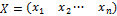as a random vector, representing n sensor signals that are observable, and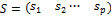as a random vector of latent mutually independent sources, where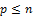. The ICA model is then given by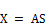Where A is a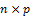matrix with full column rank, called the mixing matrix. A is assumed to be fixed, but unknown. ICA consists of estimating both the matrices A and S, when only X is known, i.e., finding a matrix W such thatHere, S is obtained by ICA based on the following two main assumptions on each source signals si in S: i) si is statistically independent of sj in S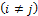, ii) si is non-Gaussian random variable.

One can recover the original sources up to a scaling and permutation provided that at most one of the underlying sources is Gaussian and the rest are non-Gaussian. Note that ideally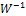should be equal to A. However,differs from A for practical cases due to limitations in ICA techniques, computational round off errors, and noise and outliers in mixed data. ICA methods find a transformation so that components extracted from mixtures are as independent as possible by maximizing or minimizing some objective function (e.g., kurtosis, entropy, negentropy, mutual information). In general, the input for ICA process is the mixed data X, while the output is the estimated source signals S. Data pre-processing' step is carried out to whiten or sphere the mixed data X. This helps in determining the number of independent components and can be carried out by classical PCA. After pre-processing steps an `optimization algorithm' based on the selected objective function, e.g. maximum likelihood estimation is employed to estimate the independent sources S from the pre-processed mixed data. Note that, in general, the order of signal sources are lost; thus, estimated S may not be identically ordered.

5. Kurtosis

The standardized fourth central moment is often regarded as the definition of kurtosis and has a history of usage for testing normality, multivariate normality and sorting independent components. Pearson  first introduced kurtosis as a measure of how flat the top of a symmetric distribution is when compared to a normal distribution of the same variance. This conventional measure can be formally defined as the standardized fourth population moment about the mean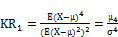Where E is the expectation operator, μ is the mean,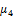is the fourth moment about the mean, and σ is the standard deviation. The normal distribution has a kurtosis of 3, so that the reference normal distribution has a kurtosis of zero. The centered conventional coefficients of kurtosis is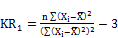Where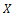bar is the sample is mean and n is the number of observations. Some uses and improvement of classical kurtosis measures refer to the literature [14,16,17]. It is well known that the sample mean is very sensitive to outliers. Since the conventional measures of kurtosis are essentially based on sample averages, they are also sensitive to outliers. Moreover, the impact of outliers is greatly amplified in the conventional measures of kurtosis due to the fact that they are raised to the third and fourth powers. The conventional measure is also not possible if only a second moment doesn’t exist of a distribution. For these reasons, we take an attempt to use quantile based kurtosis in ICA for the first time.

5.1. Quantile Kurtosis

Moors  proposed a quantile kurtosis alternative to KR1. The quantity of moors kurtosis is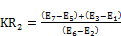Where Ei is the ith octile : that is, Ei = F-1(i/8). Moors justified this estimator on the ground that the two terms, (E7 E5) and (E3 E1), are large (small) if relatively little (much) probability mass is concentrated in the neighborhood of E6 and E2, corresponding to large (small) dispersion around μ ± σ. As we do for, KR1 we center the Moors coefficient of kurtosis at the value for the standard normal distribution. It is easy to calculate that E1 = E7 = 1.15, E2 = E6 = 0.68, E3 = E5 = 0.32 and E4 = 0 for N (0,1) and therefore the Moors coefficient of kurtosis is 1.23. Hence, the centered coefficient is given by: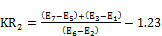One advantage of the quantile measures of kurtosis is that they are defined for a wider class of distributions than the conventional measure of kurtosis since they do not depend on the existence of the 4th moment; their resistance to outliers or robustness toward contaminating distributions are also discussed in the literature [12,13].

5.2. Role of Kurtosis in ICA

In principal component analysis, PC’s are ordered by eigen value where first eigen value is first pc, second eigen value second pc and so on. But in independent component analysis, these components have no order . For practical reasons to define a criterion for sorting these components to our interest. One measurement which can match our interest very well, is kurtosis. Kurtosis is a classical measure of non-Gaussianity, and is computationally and theoretically relatively simple. From purely Gaussian distributed data, no unique independent components can be extracted; therefore, ICA should only be applied to data sets where we can find components that have a non-Gaussian distribution. Examples of super-Gaussian distributions (highly positive kurtosis) are speech signals, because these are predominantly close to zero. However, for outlier identification super Gaussian distributions (positive kurtosis) are more interesting. Negative kurtosis can indicate a cluster structure or at least a uniformly distributed factor. Thus the components with the most negative kurtosis can give us the most relevant information. Since most negative kurtosis indicates cluster structure and highest positive kurtosis identify multivariate outlier [15, 20, 22].

6. Proposed Method

Given this emerging interest in kurtosis in independent component analysis, one should ask the following question: how useful are the measures of kurtosis used in previous empirical studies? Practically all of the previous work concerning kurtosis in ICA has used the conventional measures of kurtosis [15,20,22]. It is well known that the sample mean is very sensitive to outliers. To overcome the problem of conventional measures of kurtosis we use quantile based kurtosis. It is well known that quantile based kurtosis is robust against outlier [12,13] and take an attempt to use quantile based kurtosis to ordering independent components for multivariate outlier detection first time in ICA. The proposed method flowchart given belowFig. 1. By applying PCA for outlier detection we have to assume that the most interesting information is directly related to the highest variance in the data. Minimize the dependence using ICA then define a criterion for sorting these components to our interest using robust measure of Kurtosis.

7. Application

7.1. Real Data (Kola Data, Data Source: R)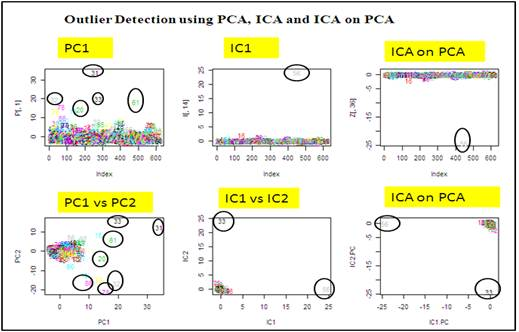Fig. 2. On the left, by applying PCA to the total data, the result is worse than the result of ICA. However, by using PCA for preprocessing before applying ICA, a more strongly outlier can be extracted. On the left, by applying PCA to the total data, the graph shows two pc’s wrongly identified more than two outlier but in IC’s, last two IC’s and ICA on PC’s gives strongly identified only two observation 33 and 56 as a outlier when IC’s ordering based on quantile measures of kurtosis.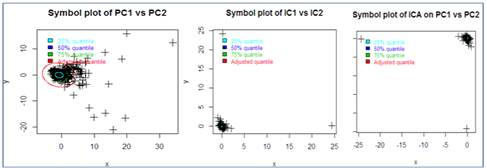Fig. 3. On the left, by applying symbol plot (25%, 50%, 75% and adjusted quantile) in PCA to the total data, first two PCA symbol plot found that at least 20 observations are outlining the adjusted quantile where in ICA and ICA on PCA only two observations are outlier that also identified in fig-2.

The Kola Data were collected in the Kola Project (1993-1998, Geological Surveys of Finland (GTK) and Norway (NGU) and Central Kola Expedition (CKE), Russia). More than 600 samples in five different layers were analyzed; this dataset contains the humus layer. A data frame with 617 observations and 44 variables. Source of data: R ("mvoutlier" package). In humus data first seven PC’s can explain 79% variability of the total variation. So we plot first PC’s that chosen according to eigen value and comparing their performance IC’s. Since IC’s has no order. For this reason we used kurtosis measure to ordering independent components where highest positive kurtosis considered IC1, second largest positive kurtoses consider IC2 and so on.

7.2. Simulation Study

In this paper we conducted a simulation study and generate 20 variables each 150 observations where each variable has 50 observation come from normal distribution with mean 100 and standard deviation (s.d) 10, 50 from normal with mean 110, s.d 5 and 50 from normal distribution with mean 112, s.d 4. According to our method, at first we apply PCA to the simulated data where 20 variables each 150 observations. We found that first 6 PC’s can explain 82% variability of the total variation, and then we apply ICA to the simulated data and ICA applying to PCA scores. The following table gives the ordering procedure of 20 independent components (IC’s). From table highest kurtosis value 27.34 found sixteen number components treated as IC1 and second largest kurtosis value extracted in 9-th components treated as IC2 and so on. Since most negative kurtosis indicates cluster structure and highest positive kurtosis identify multivariate outlier.

Tab. 1. Ordering IC’s by using quantile measure of Kurtosis (Simulated data).

 IC’s Com-1 Com-2 Com-3 Com-4 Com-5 Com-6 Com-7 Com-8 Com-9 Com-10 KR2 14.55 16.24 7.70 7.05 12.34 9.22 9.75 9.72 18.57 13.65 IC’s Com-11 Com-12 Com-13 Com-14 Com-15 Com-16 Com-17 Com-18 Com-19 Com-20 KR2 8.44 6.06 13.09 10.22 13.45 27.34 17.32 7.55 8.32 17.94 IC’s Ordering IC1 IC2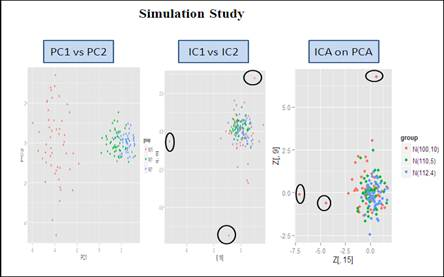Fig. 4. On the left, by applying PCA to the simulated data and plotting first two PC’s found that PC’s couldn’t detect outlier where in IC’s and PCA on ICA detect three outliers from simulated data.

8. Conclusion

In this paper we have illustrated three techniques to detect multivariate outlier detection PCA, ICA and ICA on PCA. To overcome ordering independent components we used quantile measure of kurtosis, we then apply our measure in sorting independent components in Humus data and simulated data, and try to examine the capacity of PCA, ICA and ICA on PCA for finding outliers through normal dot plot and symbol plot. In both data sets, our proposed quantile based kurtosis (Moors) ordering ICA on PCA a new visualization technique correctly diagnosis outlier than PCA. Although in our study, we considered classical method of outlier detection. In future we have to use some robust based outlier detection procedure and comparing their performance ICA based techniques.

Acknowledgment

My special gratitude goes to Prof. Dr. Mohammed Nasser for his immense and invaluable advice and guidance that contributed to the successful realization of this study. My sincere appreciation and thanks also go to the colleagues of Mathematics Department at Pabna University of Science & Technology for their unreserved knowledge sharing and cooperation.

References

1. Atkinson, A. C., Riani, M., & Cerioli, A. (2004). Exploring multivariate data with the forward search. New York: Springer-Verlag.
2. Balanda KP, MacGillivray HL (1988), Kurtosis a critical review. Journal of the American Statistical Association, 42,111-119.
3. Barnett, V, & Lewis, T. (1994). Outliers in statistical data (3rd ed.). New York: Wiley.
4. Groeneveld RA (1998) A class of quantile measures for kurtosis. Am Stat 52: 325-329.
5. Hawkins, D.M. (1980). Identification of outliers. London: Chapman and Hall.
6. Hogg, R.V. (1972),"More Light on the Kurtosis and Related Statistics," Journal of the American Statistical Association, 67, 422-424.
7. Huber PJ (1981) robust statistics. Wiley, London.
8. Hyv¨arinen, A. and Oja, E.: Independent component analysis: Algorithms and applications. Neural Networks. 4-5(13):411-430. 2000.
9. J.C. Salagubang and Erniel B. Barrios, Outlier detection in high dimensional data in the context of clustering,12th National Convention on Statistics (NCS) EDSA Shangri-La Hotel, Mandaluyong City October 1-2, 2013
10. Johnson, R. and Wischern, D. (2002). Applied Multivariate statistical analysis, 5th ed. Prentice-Hall, Inc.
11. Jones,M. and Sibson, R. What is projection pursuit? J. of the Royal Statistical Society, Ser. A, 150:1-36. 1987.
12. Kim TH, White H (2003) On more robust estimation of skewness and kurtosis: simulation and application to the S&P500 index. Department of Economics, UCSD, Paper 2003-12.
13. Kotz, S., and Seier, E. (2008), Kurtosis of the Two-Sided Power Distribution, Brazilian Journal of Probability and Statistics, 28, 6168.
14. Lihua An, S.Ejaz Ahmed. Improving the performance of kurtosis estimator. Computational Statistics and Data Analysis 52, 2669-2681. 2008.
15. Matthias Scholz, Yves Gibon, Mark Stitt and Joachim Selbig, Independent component analysis of starch deficient pgm mutants. Proceedings of the German conference on Bioinformatics. Gesellschaft fur info mark, Bonn, pp.95-104,2004.
16. Maurya V.N., Misra R.B., Jaggi C.K., and Maurya A.K., Performance analysis of powers of skewness and kurtosis based multivariate normality tests and use of extended Monte Carlo simulation for proposed novelty algorithm, American Journal of Theoretical and Applied Statistics, Science Publishing Group, USA, Vol. 4(2-1), pp. 11-18, 2015.
17. Maurya V.N., Misra R.B., Jaggi Chadra K., Maurya A.K. and Arora D.K., Design and estimate of the optimal parameters of adaptive control chart model using Markov chains technique, Special Issue: Scope of Statistical Modeling and Optimization Techniques in Management and Decision Making Process, American Journal of Theoretical and Applied Statistics, Science Publishing Group, USA, 2014.
18. Moors, J. J. A. (1988), "A Quantile Alternative for Kurtosis," The Statistician, 37, 25-32.
19. Pearson K (1905) Skew variation, a rejoinder. Biometrika 4:169212.
20. Reza, M.S., Nasser, M. and Shahjaman, M. (2011) An Improved Version of Kurtosis Measure and Their Application in ICA, International Journal of Wireless Communication and Information Systems (IJWCIS) Vol 1 No 1.
21. Rousseeuw P.J., Van Zomeren B.C. (1990). Unmasking multivariate outliers and leverage points. Journal of the American Statistical Association. Vol. 85(411), pp. 633-651.
22. Scholz, M., Gatzek, S., Sterling, A., Fiehn, O., and Selbig, J. Metabolite fingerprinting: detecting biological features by independent component analysis. Bioinformatics 20, 2447-2454, 2004.

 Contents 1. 2. 3. 4. 5. 5.1. 5.2. 6. 7. 7.1. 7.2. 8.
Article ToolsAbstractPDF(323K)